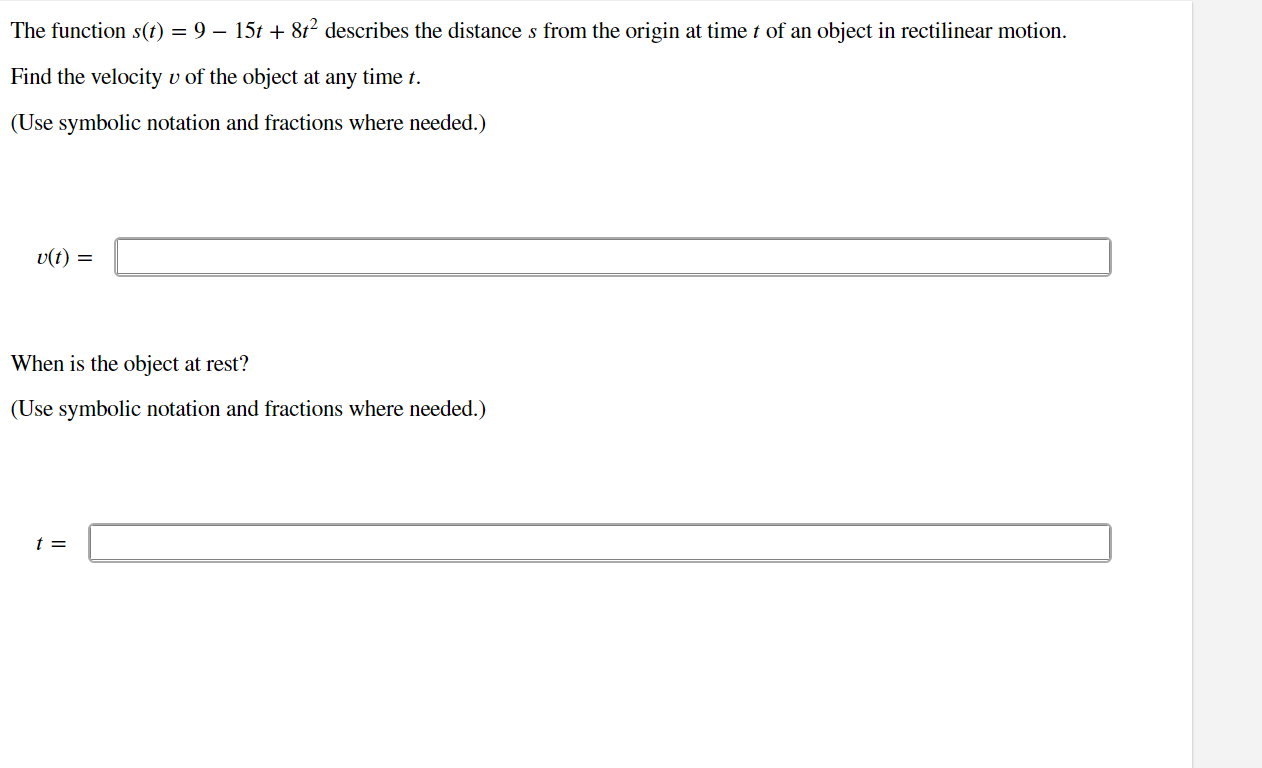# Question Solved1 AnswerThe function 𝑠(𝑡)=9−15𝑡+8𝑡2 describes the distance 𝑠 from the origin at time 𝑡 of an object in rectilinear motion. Find the velocity 𝑣 of the object at any time 𝑡. The function s(t) = 9 – 15t + 8t² describes the distance s from the origin at time t of an object in rectilinear motion. Find the velocity v of the object at any time t. (Use symbolic notation and fractions where needed.) v(t) = When is the object at rest? (Use symbolic notation and fractions where needed.) t =The function 𝑠(𝑡)=9−15𝑡+8𝑡2 describes the distance 𝑠 from the origin at time 𝑡 of an object in rectilinear motion. Find the velocity 𝑣 of the object at any time 𝑡.Transcribed Image Text: The function s(t) = 9 – 15t + 8t² describes the distance s from the origin at time t of an object in rectilinear motion. Find the velocity v of the object at any time t. (Use symbolic notation and fractions where needed.) v(t) = When is the object at rest? (Use symbolic notation and fractions where needed.) t =
More
Transcribed Image Text: The function s(t) = 9 – 15t + 8t² describes the distance s from the origin at time t of an object in rectilinear motion. Find the velocity v of the object at any time t. (Use symbolic notation and fractions where needed.) v(t) = When is the object at rest? (Use symbolic notation and fractions where needed.) t =